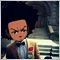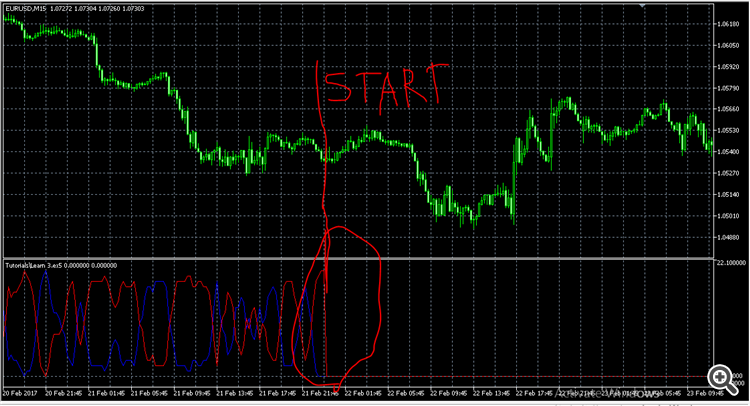My MT5 Indicator does not update in BackTest44332

i made a custom indicator in MT5, it works well on live or demo chart but when i backtest, it just stops updating from current candle and remains at 0 till end of test

but all previous candles will have values for the indicatorin the image above, test started at that red line

for(int i=prev_calculated;i<rates_total-100;i++){
x=0;
y=0;

ArraySetAsSeries(close,true);
ArraySetAsSeries(ExtBuffer1,true);
ArraySetAsSeries(ExtBuffer2,true);

if(close[i]>close[i+1])x++;
if(close[i]<close[i+1])y++;

if(close[i]>close[i+2])x++;
if(close[i]<close[i+2])y++;

//.........................................Identical Codes

if(close[i+4]>close[i+6])x++;
if(close[i+4]<close[i+6])y++;

ExtBuffer1[i]=MathAbs(x);
ExtBuffer2[i]=MathAbs(y);

}